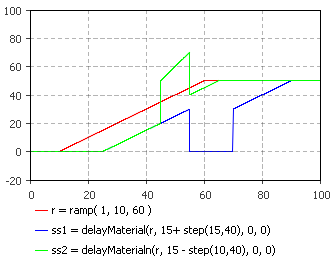AnyLogic

# delayMaterial

delayMaterial is the system dynamics function that returns the value of the input delayed by the delay time.

The initial value is the value of the variable on the left-hand side of the equation at the start of the simulation. The delay time can be a variable. If delay time is decreasing, some values of the input will be added to more recent inputs for output. If delay time is increasing, missingValue will be used when no output is available at a time. This function is particularly useful for modeling queues and production processes with varying and often random processing times.

The plot below illustrates how the function works:This function can be called in formulas of system dynamics variables and has the following notation:

• delayMaterial(flow, delayTime, initialValue, missingValue)

flow can be a flow variable, or a numeric expression of any complexity.

delayTime can be either a constant or a numeric expression (e.g. a function call, or a numeric parameter).

initialValue — initial value.

missingValue — this value is used when delay time is increasing and no output is available at a time.

#### Units

flow — unit

delayTime — time

initialValue — unit

missingValue — unit

delayMaterial() — unit

The output units are the same as the input ones.